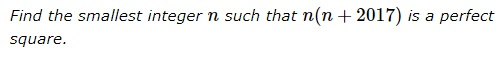# A Farewell Problem for the Year 2017

### Problem### Solution 1

For an odd $n,$ say $n=2m-1,$ $\displaystyle\sum_{k=1}^m(2k-1)=m^2.$ Thus,

$\displaystyle 1+3+\ldots+2015=\sum_{k=1}^{1008}(2k-1)=1008^2\\ \displaystyle 1+3+\ldots+2015+2017=1008^2+2017=1009^2.$

Thus taking $n=1008^2,$ $n(n+2017)=(1008\cdot 1009)^2.$

There are no other solutions. Indeed, if $n$ is not a perfect square, it is bound to have a factor that is shared with $n+2017$ and, hence, with $2017.$ But the latter is prime so that the only possibility is $n=s^2\cdot 2017,$ implying $n(n+2017)=(2017s)^2(s^2+1)$ that could not be perfect square unless $s=0.$

It follows that both $n$ and $n+2017$ are bound to be perfect squares, say $n+a^2,$ $n+2017=b^2.$ From this $(b-a)(b+a)=2017$ and, subsequently, $b=1009,$ $a=1008.$

### Solution 2

Let $n(n+2017)=k^2$ ($k\in\mathbb{N}$). Hence, $(k-n)(k+n)=2017n$. $2017$ is prime. Thus, $2017|(k-n)$ or/and $2017|(k+n)$. Suppose $k-n=2017p$ ($p\in\mathbb{N})$). Then, \begin{equation*} k+n=\frac{n}{p} \Rightarrow k=n\left(\frac{1-p}{p}\right). \end{equation*} Thus, $p\leq 1$ and this option is not possible. Alternatively, let $k+n=2017p$ ($p\in\mathbb{N}$). Thus, \begin{eqnarray*} k+n&=&2017p \\ k-n&=&\frac{n}{p}. \end{eqnarray*} For $k$ to be integral, $p|n$. Eliminating, $k$, \begin{equation*} n=\frac{2017p^2}{2p+1}. \end{equation*} Suppose $(2p+1)|2017$. The smallest $p$ satisfying this corresponds to $2p+1=2017$ or $p=1008$. Note, in this case $\boldsymbol{n}=p^2=1008^2=\boldsymbol{1016064}$ and $k=p+n=1008+1016064=1017072$. The remaining option is that $(2p+1) \hspace{-4pt}\not|\hspace{2pt} 2017$ and $(2p+1)|p^2$. Suppose $p^2=(2p+1)m$ ($m\in\mathbb{N}$). The only positive root for this quadratic equation in $p$ is \begin{equation*} p=m+\sqrt{m(m+1)}. \end{equation*} However, the product of two consecutive natural numbers cannot be a square as $m^2\lt m(m+1)\lt (m+1)^2$. Thus, this option has no integral solution for $p$ and thus $n$.

### Acknowledgment

The problem with $2013$ instead of $2017$ was offered at the 2013 Pan African Mathematics Olympiad. It was published at the Crux Methematicorum as problem CC151 (2016), with two solutions. Solution 1 combines elements of both; Solution 2 is by Amit Itagi.